# Transformations

Transformations

In the 19th century, Felix Klein proposed a new perspective on geometry known as transformational geometry. Most of the proofs in geometry are based on the transformations of objects.

In this chapter, you will learn more about transformations, and once your basics are in order, will also be introduced to transformations of function rules, transformations of quadratic functions, types of transformations, transformations definition, and transformations algebra.

Check out the interactive examples to know more about the lesson and try your hand at solving a few interesting practice questions at the end of the page.

## Lesson Plan

 1 What Are Transformations? 2 Tips and Tricks 3 Important Notes on Transformations 4 Solved Examples on Transformations 5 Interactive Questions on Transformations

## What Are Transformations?

A function f that maps to itself is called the transformation .i.e., $$f : X \rightarrow X$$

The preimage $$X$$ becomes the image $$X$$ after the transformation.

### Transformations in Math

Transformations in Math describe how two-dimensional figures move around a coordinate plane.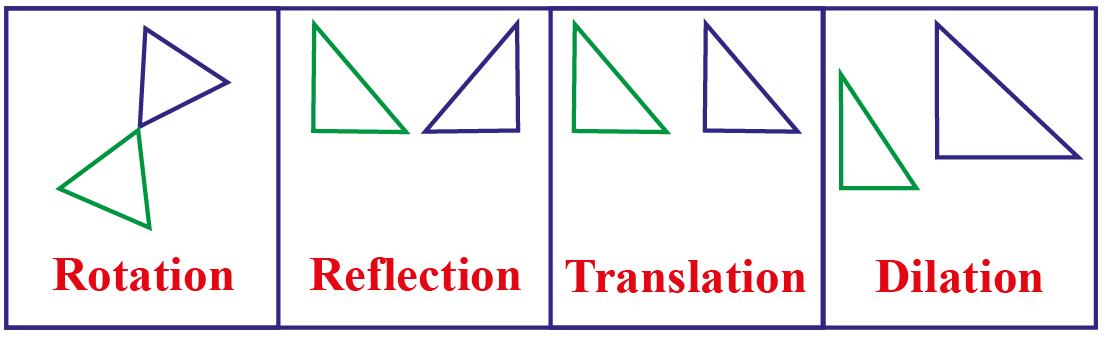## What Are The Common Types of Transformations?

There are four common types of transformations - translation, rotation, reflection, and dilation.

From the transformations definition, we have: rotation about any point, reflection over any line, and translation along any vector.

These are rigid transformations wherein the image is congruent to its preimage.

Dilation is performed about any point. Here the image is similar to its preimage.

Transformation Function Result
Rotation Rotates or turns the preimage around an axis No change in size or shape
Reflection Flips the preimage and produces the mirror-image No change in size or shape or orientation
Translation Slides or moves the preimage No change in size or shape; Changes only the direction of the shape
Dilation Stretches or shrinks the preimage Expands or contracts the shape

### Types of Transformation

In the following simulation, observe the transformations of the preimage to the image by adjusting the slider and the points.Tips and Tricks
• We can use the formula of transformations in graphical functions to obtain the graph just by transforming the basic or the parent function, and thereby move the graph around, rather than tabulating the coordinate values.
• Transformations help us visualize and learn the equations in algebra.

## What is The Rule for Transformations?

Consider a function $$f(x)$$.

On a coordinate grid, we use the x-axis and y-axis to measure the movement.

Here are the transformations of function rules that could be applied to the graphs of functions.

### Transformations Algebra

Transformations can be represented algebraically.

• Let us consider an example of a transformation of translation.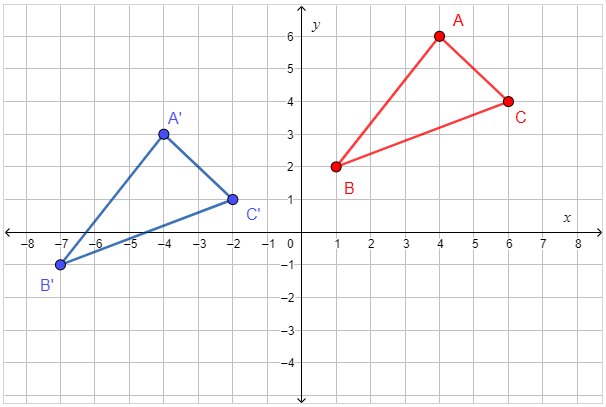To describe the position of the blue figure relative to the red figure, let’s observe the relative positions of their vertices.

We need to find the positions of  A′,B′, and C′ comparing its position with respect to the points A, B, and C.

We find that, A′,B′, and C′ are:

• 8 units to the left of A,B, and C respectively.
• 3 units below A,B, and C respectively.

This translation can algebraically be translated as

8 units left and 3 units down.

i.e.$$(x,y) \rightarrow (x-8, y-3)$$

We can apply the transformation rules to graphs of functions.

• Here is the graph of a function that shows the transformation of reflection.

The first picture shows the function $$f(x) = x^3$$.

The transformation $$g(x) = - x^3$$ is done and it fetches the reflection of the f(x) about the x-axis.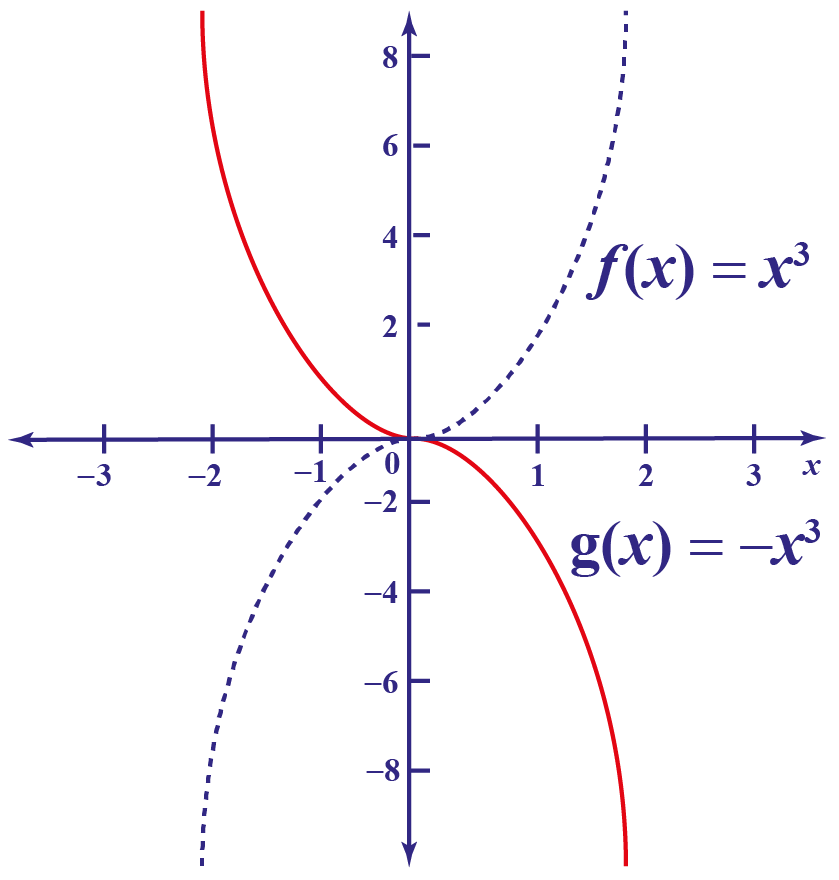• Next, let us witness the transformation of translation.

This preimage in the first function shows the function $$f(x) = x^2$$

The transformation $$f(x) = (x+2)^2$$ shifts the parabola 2 steps right.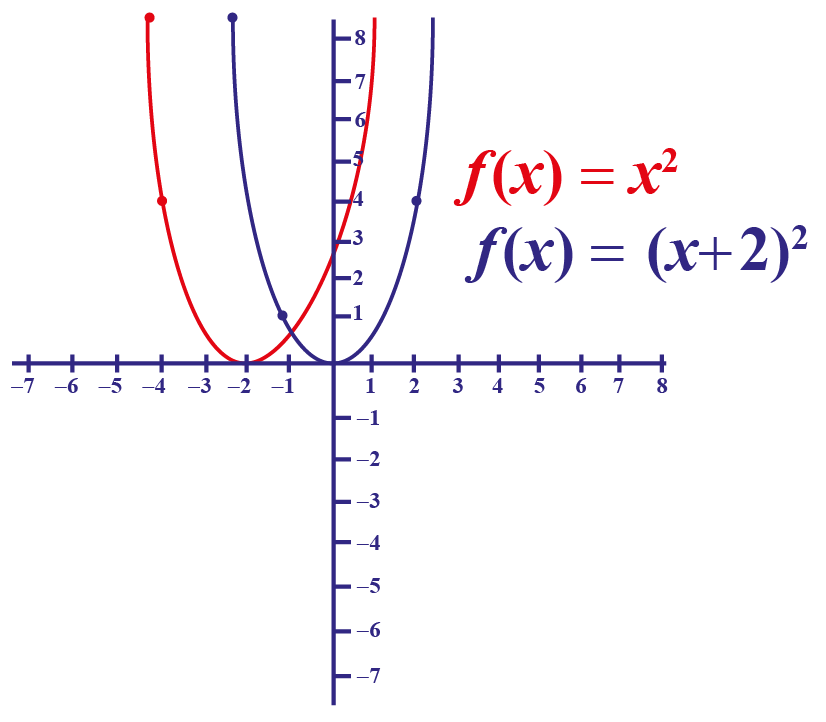• In the function graph below, we observe the transformation of rotation.

To rotate 90$$^\circ$$: $$(x,y)\rightarrow (-y, x)$$

To rotate 180$$^\circ$$: $$(x,y) \rightarrow (-x,-y)$$

To rotate 270$$^\circ$$: $$(x,y) \rightarrow (y, -x)$$

Here we see that the preimage is rotated to 180$$^\circ$$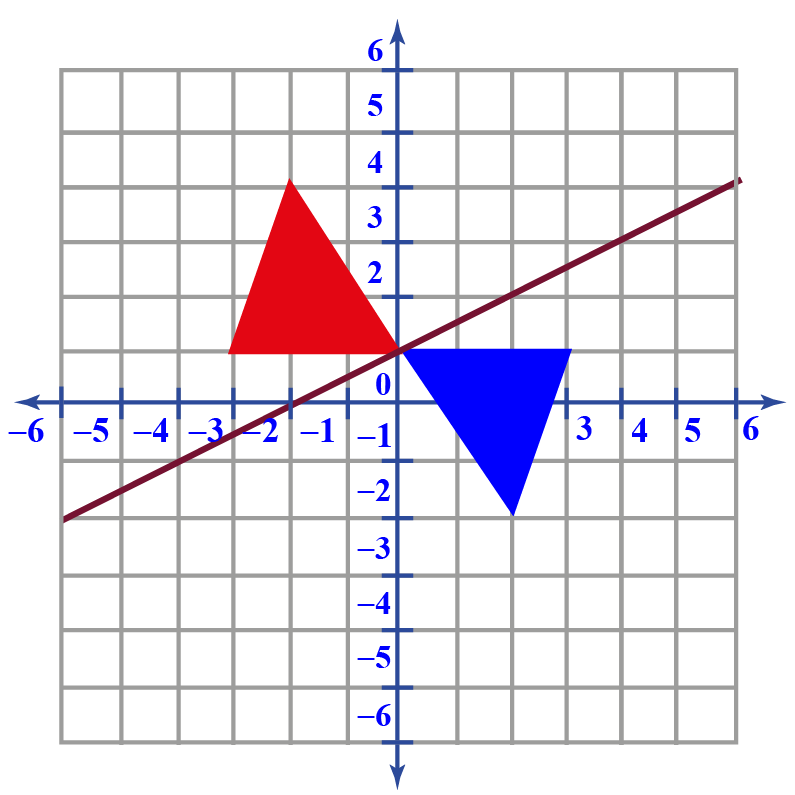## Formula of Transformations

Let us consider the graph $$f(x) = x^2$$

• Suppose we need to graph $$f(x) = x^2 -3$$, we shift the vertex 3 units down.
• Suppose we need to graph $$f(x) = 3x^2+ 2$$, we shift the vertex two units up and stretch vertically by a factor of three.
• Suppose we need to graph $$f(x) = 2(x-1) ^2$$, we shift the vertex one unit to the right and stretch vertically by a factor of 2
• Thus, we get the general formula of transformations as
 $$f(x) =a(bx-h)^n+k$$
• where k is the vertical shift,
• h is the horizontal shift,
• a is the vertical stretch and
• b is the horizontal stretch.

Likewise, $$f(x)$$ can be transformed in many ways.

This table shows the resultant graph after the transformation applied on $$f(x)$$.

Transformation of $$f(x)$$ Transformation of graph Change $$(x,y)$$ to
$$-f(x)$$ reflect f(x) over the x-axis $$(x,-y)$$
$$f(-x)$$ reflect f(x) over the y-axis $$(-x,y)$$
$$f(x)+a$$ shift f(x) up by a units $$(x,y+a)$$
$$f(x) - a$$ shift f(x) down by a units $$(x,y-a)$$
$$f(x+a)$$ shift f(x) left by a units $$(x-a,y)$$
$$f(x-a)$$ shift f(x) right by a units $$(x+a,y)$$
$$a\dot f(x)$$ stretch f(x) vertically $$(x,ay)$$
$$f(ax)$$ stretch f(x) horizontally $$(\dfrac{x}{a},y)$$Important Notes
• Transformations in geometry can be combined. A shape can be reflected or rotated or translated or dilated or can have a combination of these transformations.
• Transformations are expressed algebraically in the graph functions.

## Solved Examples

 Example 1

How can Mike describe this translation algebraically?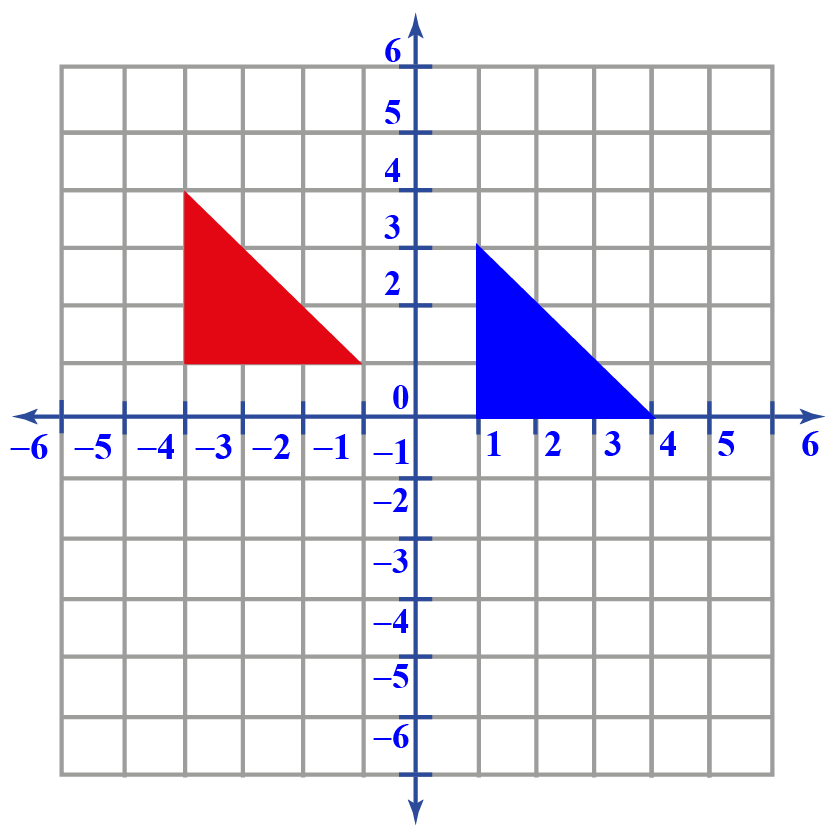Solution

Let the red triangle be the preimage and the blue triangle be the transformed image.

Represent it on the coordinate plane as $$(x,y)$$

Comparing the relative positions of the triangles, we can observe that the blue triangle is placed one position down and 5 positions right.

Thus, the transition is expressed algebraically as $$(x+5, y-1)$$

 $$\therefore$$, $$(x,y) \rightarrow (x+5, y-1)$$
 Example 2

How has this figure been transformed?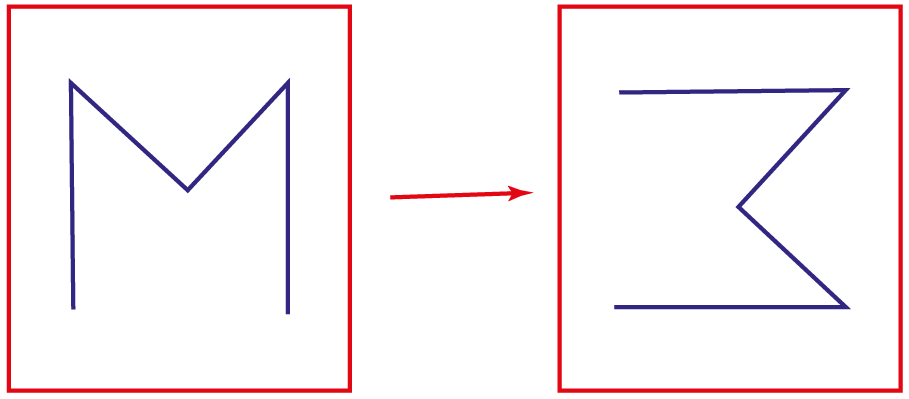Solution

The rotation of an image turns its position around an axis.

Thus, here we can observe that M is rotated 90$$^\circ$$ clockwise.

 $$\therefore$$, the transformation of rotation can be observed in the figure.
 Example 3

A point A(1, 3) is translated 4 units to the right.

What are the coordinates of the transformed image?

Solution

Let A be the preimage.

A' be the image.

If the preimage is to be moved right, then the x-coordinate undergoes a change of 4 units.

$$A \rightarrow$$ A' = (1,3)\rightarrow ((1+4),3)\)

$$A \rightarrow$$ A' = (1,3) \rightarrow (5,3)\)

 $$\therefore$$ The coordinates of the transformed image = (5,3)
 Example 4

What do the following transformations do to the graph?

i) $$f(x) \rightarrow f(x) - 2$$

ii) $$f(x) \rightarrow f(x-2)$$

Solution

$$f(x) \rightarrow f(x) - 2$$

The y-coordinate undergoes the change.

Thus, the transformation here is translation 2 units down.

$$f(x) \rightarrow f(x-2)$$

The x-coordinate undergoes the change.

Thus, the transformation here is translation 2 units right.

 $$\therefore$$ i) Translation is 2 units down ii)Translation is 2 units right

## Interactive Questions

Here are a few activities for you to practice. Select/Type your answer and click the "Check Answer" button to see the result.

## Let's Summarize

We hope you enjoyed learning about transformations with the simulations and practice questions. Now, you will be able to easily solve problems on transformations of function rules, transformations of quadratic functions, types of transformations, transformations definition, and transformations algebra.

At Cuemath, our team of math experts is dedicated to making learning fun for our favorite readers, the students!

Through an interactive and engaging learning-teaching-learning approach, the teachers explore all angles of a topic.

Be it worksheets, online classes, doubt sessions, or any other form of relation, it’s the logical thinking and smart learning approach that we, at Cuemath, believe in.

## 1. Why are transformations important in Math?

Math is about identifying patterns and understanding the relationships between concepts to work out a solution to a problem.

Transformations are important in Math to mainly know the congruence and similarity of figures in a plane.

## 2. How do you plot a reflection?

When we reflect a point across the x-axis, the y-coordinate is transformed and the x-coordinate remains the same.

x-coordinate will have the same sign, but the y-coordinate's sign changes.

More Important Topics
Numbers
Algebra
Geometry
Measurement
Money
Data
Trigonometry
Calculus
More Important Topics
Numbers
Algebra
Geometry
Measurement
Money
Data
Trigonometry
Calculus